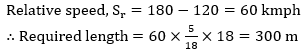# Set-27 Arithmetic (Miscellaneous) For SBI PO and SBI Clerk 2019 | Must Go Through These QuestionsDear Aspirants,
We are providing the most important Arithmetic (Miscellaneous) Questions for SBI PO 2019, SBI Clerk 2019 and all other competitive bank and insurance exams. These questions have very high chances to be asked in SBI PO 2019, SBI Clerk 2019.

1. Mukesh sold a cow to Rakesh at 20% profit. Rakesh sold it to Mahesh at 25% profit. Mahesh sold it to Mona at 10% profit. If Mona paid Rs. 990 for the cow then at what price Mukesh purchased it ?

2. A hockey team has 14 players including two goalkeepers. In how many ways can playing eleven be selected ?

3. A group of 15 members have the average age of 15 years. First 5 members among them have the average age of 14 years and after that 9 members have the average age of 16 years. What is the age of 15th member?

4. A committee of 5 persons is to be formed from 5 men and 4 women. What is the Probability of having at least 3 men in the committee ?

5. If a person reduces his speed by 2/3 of his normal speed then he takes one hour more in walking certain distance. The time (in hours) to cover the distance with normal speed is

6. A person left Rs. 8,600 for his 5 sons, 4 daughters and 2 nephews. If amount received by each daughter is four times that of each nephew and amount received by each son is five times that of each nephew, then what will be the share of each daughter ?

7. A sum of money amounts to Rs. 6,000 in 5 years and to Rs. 8,000 in 10 years at a compound interest rate per annum. What is the sum?

8. It takes 8 hours to fill a tank in normal condition but due leakage in the bottom of the tank it take 2 hours more. If the tank is completely filled then how much time it will take in emptying the tank due to leakage ?

9. An article was sold for Rs. 144. If the percentage of profit was numerically equal to the cost price, then what is the cost of article ?

10. Two trains are travelling in same direction with speeds 120 kmph and 180 kmph respectively. The faster train completely passes a man sit in the slower train in 18 seconds. Find the length of the faster train.

1. Mukesh sold a cow to Rakesh at 20% profit. Rakesh sold it to Mahesh at 25% profit. Mahesh sold it to Mona at 10% profit. If Mona paid Rs. 990 for the cow then at what price Mukesh purchased it ?

Ans:3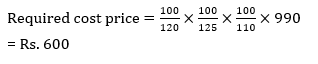2. ##### 2. Question

A hockey team has 14 players including two goalkeepers. In how many ways can playing eleven be selected ?

Ans:2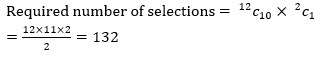3. ##### 3. Question

A group of 15 members have the average age of 15 years. First 5 members among them have the average age of 14 years and after that 9 members have the average age of 16 years. What is the age of 15th member?

Ans:1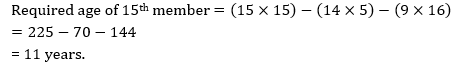4. ##### 4. Question

A committee of 5 persons is to be formed from 5 men and 4 women. What is the Probability of having at least 3 men in the committee ?

Ans: 4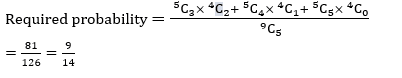5. ##### 5. Question

If a person reduces his speed by 2/3 of his normal speed then he takes one hour more in walking certain distance. The time (in hours) to cover the distance with normal speed is

Ans:1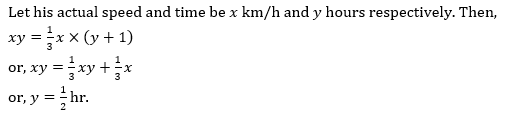6. ##### 6. Question

A person left Rs. 8,600 for his 5 sons, 4 daughters and 2 nephews. If amount received by each daughter is four times that of each nephew and amount received by each son is five times that of each nephew, then what will be the share of each daughter ?

Ans:3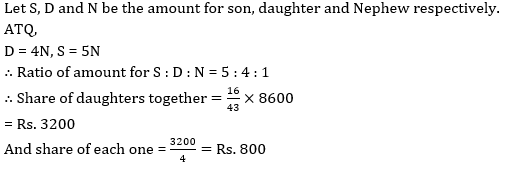7. ##### 7. Question

A sum of money amounts to Rs. 6,000 in 5 years and to Rs. 8,000 in 10 years at a compound interest rate per annum. What is the sum?

Ans:3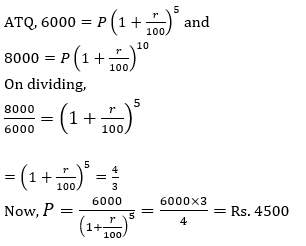8. ##### 8. Question

It takes 8 hours to fill a tank in normal condition but due leakage in the bottom of the tank it take 2 hours more. If the tank is completely filled then how much time it will take in emptying the tank due to leakage ?

Ans:3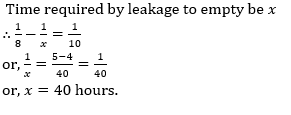9. ##### 9. Question

An article was sold for Rs. 144. If the percentage of profit was numerically equal to the cost price, then what is the cost of article ?

Ans:4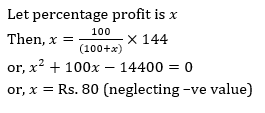10. ##### 10. Question

Two trains are travelling in same direction with speeds 120 kmph and 180 kmph respectively. The faster train completely passes a man sit in the slower train in 18 seconds. Find the length of the faster train.

Ans:3